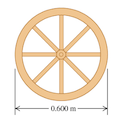Physics Practice Problems Moment of Inertia of Systems Practice Problems Solution: A wagon wheel is constructed as shown in the figur...

🤓 Based on our data, we think this question is relevant for Professor Brar & Pan's class at UW-MADISON.

# Solution: A wagon wheel is constructed as shown in the figure. The radius of the wheel is 0.300 m, and the rim has mass 1.41 kg. Each of the eight spokes, that lie along a diameter and are 0.300 m long, has mass 0.260 kg. What is the moment of inertia of the wheel about an axis through its center and perpendicular to the plane of the wheel?

###### Problem
A wagon wheel is constructed as shown in the figure. The radius of the wheel is 0.300 m, and the rim has mass 1.41 kg. Each of the eight spokes, that lie along a diameter and are 0.300 m long, has mass 0.260 kg. What is the moment of inertia of the wheel about an axis through its center and perpendicular to the plane of the wheel?Moment of Inertia of Systems

Moment of Inertia of Systems

#### Q. A uniform bar has two small balls glued to its ends. The bar is 2.00 m long and has mass 6.00  kg , while the balls each have mass 0.300 kg and can be...

Solved • Mon Mar 18 2019 16:35:14 GMT-0400 (EDT)

Moment of Inertia of Systems

#### Q. A thin uniform rod 50.0 cm long and with mass 0.320 kg is bent at its center into a V shape, with a 70.0 degree angle at its vertex. Find the moment o...

Solved • Mon Mar 18 2019 16:35:05 GMT-0400 (EDT)

Moment of Inertia of Systems

#### Q. A sphere consists of a solid wooden ball of uniform density 800kg/m3 and radius 0.30 m and is covered with a thin coating of lead foil with area densi...

Solved • Mon Mar 18 2019 16:35:03 GMT-0400 (EDT)

Moment of Inertia of Systems

#### Q. A thin, flat, uniform disk has mass M and radius R. A circular hole of radius R/4, centered at a point R/2 from the disks center, is then punched in t...

Solved • Mon Mar 18 2019 16:35:03 GMT-0400 (EDT)